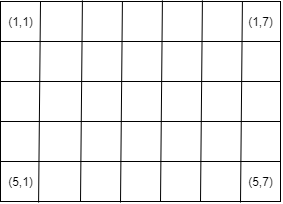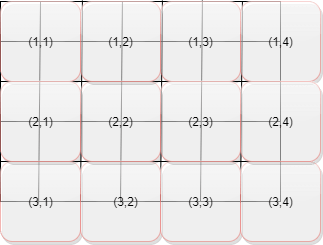# sub2blocksub

Convert pixel subscripts to block subscripts

## Syntax

``blocksub = sub2blocksub(bim,pixelsub)``
``blocksub = sub2blocksub(bim,pixelsub,'Level',L)``

## Description

````blocksub = sub2blocksub(bim,pixelsub)` converts the pixel subscripts in `pixelsub` to the block subscripts identifying the block containing the specified pixel. ```

example

````blocksub = sub2blocksub(bim,pixelsub,'Level',L)` additionally specifies the resolution level to use in a multi-resolution image. By default, `Level` is `1`.```

## Examples

collapse all

Create a small sample image as a 5-by-7 matrix of zeros. Here is an illustration of the small sample image, with the pixel coordinates of the four corners provided.Create a blocked image from the sample image, specifying a 2-by-2 block size. To create this blocked image, use `blockedImage` in write mode.

`bim = blockedImage([], [5 7], [2 2], uint8(0), "Mode", 'w');`

Here is an illustration of the blocked image overlaid on the original image. It is divided into 2-by-2 blocks. In the diagram, each block contains it's block coordinates.To determine which block contains a particular pixel, convert the pixel subscripts into block subscripts by using the sub2`blocksub` function. By default, if the image is a multiresolution image, sub2`blocksub` uses pixel coordinates from coarest level, although you can specify any level. Since the sample image has only one resolution level, sub2`blocksub` converts level 1.

`[blocksub] = sub2blocksub(bim,[2 3])`
```blocksub = 1×2 1 2 ```

## Input Arguments

collapse all

Blocked image, specified as a `blockedImage` object.

Pixel subscripts, specified as a K-by-N integer-valued vector, N is the number of dimensions and K is the number of coordinates.

Example:

Data Types: `single` | `double` | `int8` | `int16` | `int32` | `int64` | `uint8` | `uint16` | `uint32` | `uint64` | `logical`

## Output Arguments

collapse all

Subscripts of the block that contains the pixel, returned as a K-by-N integer-valued vector, for an N-dimensional blocked image. K is the number of coordinates.